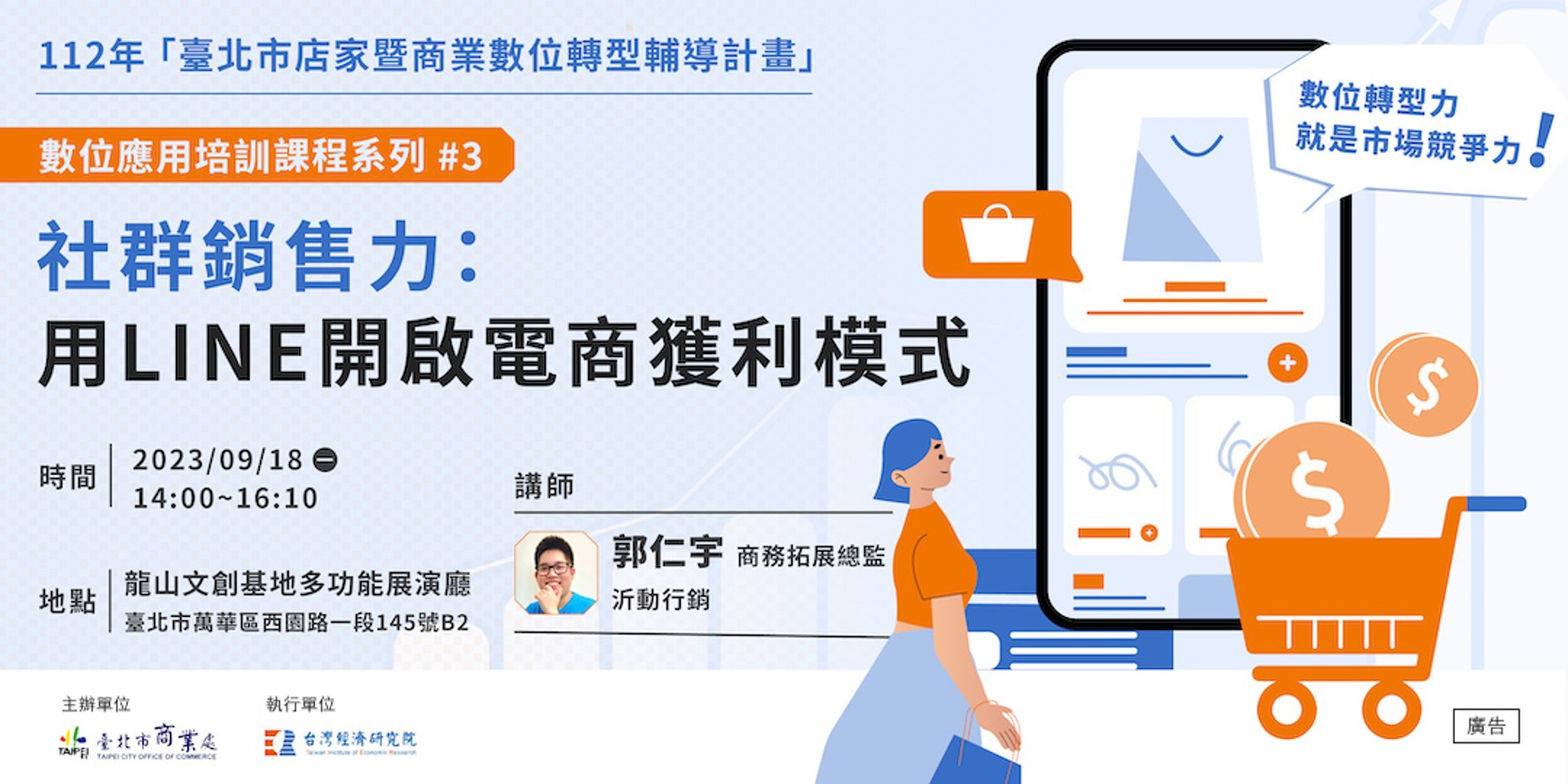# 免「仲介」　OpenVINO能直接支援PyTorch模型物件！

### OpenVINO直接支援PyTorch模型物件

```import torchvision
import torch
from openvino.tools.mo import convert_model

model = torchvision.models.resnet50(pretrained=True)
ov_model = convert_model(model)
```• torch.nn.Module
• torch.jit.ScriptModule
• torch.jit.ScriptFunction

```import torchvision
import torch
from openvino.tools.mo import convert_model

model = torchvision.models.resnet50(pretrained=True)
ov_model = convert_model(model, example_input=torch.zeros(1, 3, 100, 100))
```

• openvino.runtime.Tensor
• torch.Tensor
• np.ndarray
• list or tuple with tensors (openvino.runtime.Tensor / torch.Tensor / np.ndarray)
• dictionary where key is the input name, value is the tensor (openvino.runtime.Tensor / torch.Tensor / np.ndarray)

```serialize(ov_model, str(ir_model_xml))
```

### BERT 模型案例實戰

1. 獲取 PyTorch 模型物件

```torch_model =
BertForSequenceClassification.from_pretrained(PRETRAINED_MODEL_DIR)
```

2. 設置模型參數並轉化為OpenVINO模型物件

```input_shape = PartialShape()
input_shape, np.int64),("token_type_ids", input_shape, np.int64)]
default_input = torch.ones(1, MAX_SEQ_LENGTH, dtype=torch.int64)
inputs = {
"input_ids": default_input,
"token_type_ids": default_input,
}
model = convert_model(torch_model, example_input=inputs, input=input_info)

3. 準備校驗資料集，並啟動量化```

```calibration_dataset = nncf.Dataset(data_source, transform_fn)
# Quantize the model. By specifying model_type, we specify additional
transformer patterns in the model.
quantized_model = nncf.quantize(model, calibration_dataset,
model_type=ModelType.TRANSFORMER)
```

4. 編譯量化後的模型物件，並進行推理

```compiled_quantized_model = core.compile_model(model=quantized_model, device_name="CPU")
output_layer = compiled_quantized_model.outputs
result = compiled_quantized_model(inputs)
result = np.argmax(result)
print(f"Text 1: {sample}")
print(f"Text 2: {sample}")
print(f"The same meaning: {'yes' if result == 1 else 'no'}")
```

```Text 1: Wal-Mart said it would check all of its million-plus domestic workers to ensure they were legally employed .
Text 2: It has also said it would review all of its domestic employees more than 1 million to ensure they have legal status .
The same meaning: yes
```

### 立即加入會員！ 全站文章無限看~

###### 會員福利
1

2

3

4Author: 楊亦誠